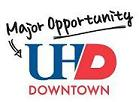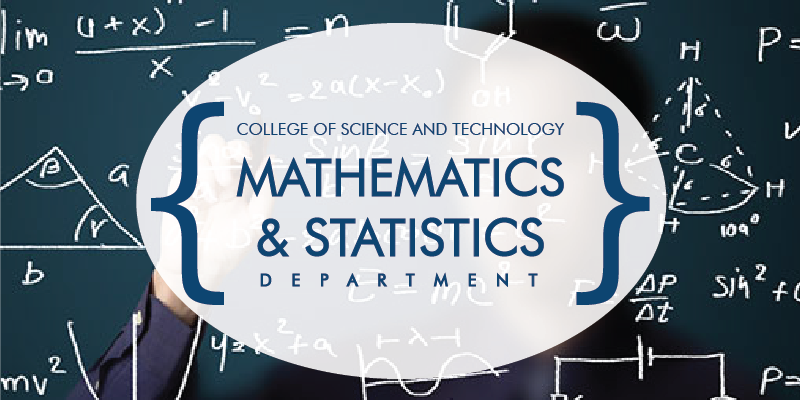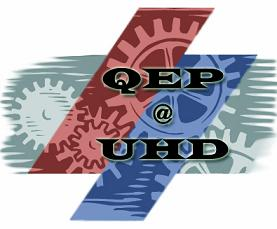## UHD Algebra Student Web Page

Welcome to the University of Houston-Downtown Algebra Student Web Page. This is where you can find additional resources and links to help you succeed in MATH 0300, 1300, 1301, 1310, and other courses too. Use the following anchor links to quickly navigate to the appropriate section of this web page:

Watch streaming video of a MATH 1301 Final Review Session on YouTube!

MATH 0300: Beginning Algebra

Prerequisite: A TSIA2 score that does not meet college readiness in Mathematics, and co-enrollment in MATH 1310 or STAT 1312. Successful completion of MATH 1310 or STAT 1312 (with a C or better) will satisfy the TSI requirements for developmental mathematics. For current TSIA2 College Readiness scores, please click here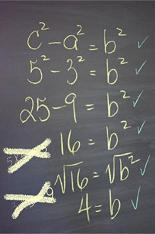Catalog Description:This course is intended to build and reinforce the essential geometry, arithmetic and algebra skills needed for Intermediate Algebra (MATH 1300). Topics include real numbers and their properties, order of operations and simplifying real-valued expressions, simplifying algebraic expressions, integer exponents, solving linear equations and inequalities, an introduction to the Cartesian coordinate system, systems of first degree equations in two variables solved by graphical and algebraic methods and word problems. This course may not be used to satisfy degree requirements.

Resources:

MATH 1300: Intermediate Algebra

Prerequisite: A TSIA2 score that does not meet college readiness in Mathematics, and co-enrollment in MATH 1301 or MATH 1324. Successful completion of MATH 1301 or MATH 1324 (with a C or better) will satisfy the TSI requirements for developmental mathematics. For current TSIA2 College Readiness scores, please click here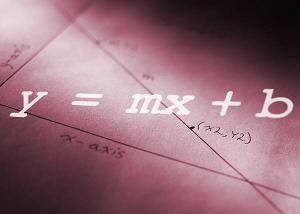Catalog Description:A survey of pre-college algebra intended to build and reinforce the essential algebra skills needed for College Algebra (MATH 1301). Topics include operations with algebraic expressions, factoring, the Cartesian coordinate system, lines, slope and distance formulas, integer and rational exponents, radicals, an introduction to quadratic equations, an introduction to functions and word problems. This course may not be used to satisfy degree requirements.

Resources:

MATH 1301: College Algebra

Prerequisite: A grade of C or better in MATH 1300, or TSIA2 score meeting college readiness in Mathematics, or TSIA2 MATH complete, or TSIA2 MATH exempt. For current TSIA2 College Readiness scores, please click here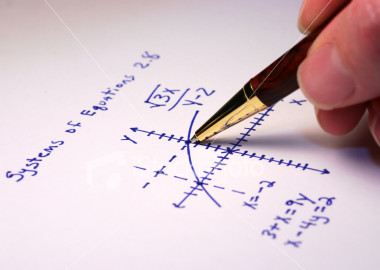Catalog Description: College-level topics in algebra including variation, systems of equations, nonlinear inequalities, functions and their graphs, lines, quadratic equations and functions, complex numbers, polynomials, exponential and logarithmic functions, the algebra of functions, and applications related to these topics.

Resources:

Tools:

MATH 1310: Contemporary Mathematics

Prerequisite: A grade of C or better in MATH 0300, or TSIA2 score meeting college readiness in Mathematics, or TSIA2 MATH complete, or TSIA2 MATH exempt. For current TSIA2 College Readiness scores, please click here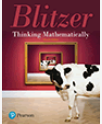Catalog Description:An introduction to contemporary mathematical ideas and problem-solving techniques. Designed for students requiring one college-level mathematics course. This course cannot be applied toward any degree in the Department of Mathematics and Statistics.

Resources:

Trig Preliminaries for Calculus

This section contains the Trigonometry Preliminaries for Calculus review worksheet, which you can download here. Experience has shown that many students in Calculus may have trouble because their trigonometry skills have gotten rusty. The purpose of this worksheet is to help you review the trigonometry skills needed for Calculus.

How to Get the Most Out of Studying

The following series of five short videos show how to study effectively in college.